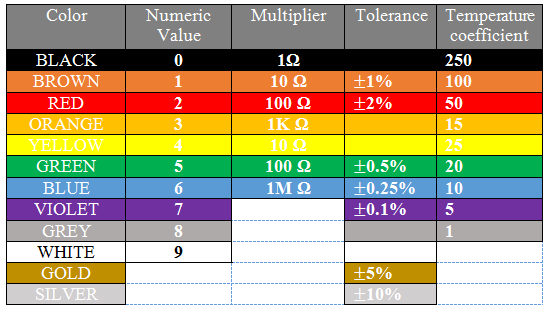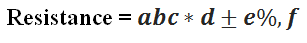# 6-Band Resistor Color Code Calculator

 First Digit Second Digit Third Digit Multiplier Tolerance Temp. Coefficient 1 Brown 2 Red 3 Orange 4 Yellow 5 Green 6 Blue 7 Violet 8 Gray 9 White 0 Black 1 Brown 2 Red 3 Orange 4 Yellow 5 Green 6 Blue 7 Violet 8 Gray 9 White 0 Black 1 Brown 2 Red 3 Orange 4 Yellow 5 Green 6 Blue 7 Violet 8 Gray 9 White x1 Black x10 Brown x100 Red x1000 Orange x10000 Yellow x100000 Green x1000000 Blue x10M Violet x100M Gray x1G White x0.1 Gold x0.01 Silver ± 1% Brown ± 2% Red ± 3% Orange ± 4% Yellow ± 0.5% Green ± 0.25% Blue ± 0.10% Violet ± 0.05% Gray ± 5% Gold ± 10% Silver 100 Brown 50 Red 15 Orange 25 Yellow 10 Blue 5 Violet Resistance : Tolerance : Temp. Co. :

A resistor is identified by its pattern of color of bands. There are 4, 5 and 6 band resistors. To calculate the resistance of a resistor, you can select the appropriate color bands in the above resistor color code calculator.

### How to calculate the resistance?

Just have a look to the resistor color code chart below and see the calculations, how the resistance value is calculated according to this chart.### Calculating resistance for a 6-band resistora’ represents the 1st significant digit, which is the first band color of resistor.

b’ represents the 2nd  significant digit, which is the second band color of resistor.

c’ represents the 3rd   significant digit, which is the third band color of resistor.

d’ represents the 4th   significant digit, which is the fourth band color of resistor and this is the multiplier value used in formula.

e’ represents the 5th  significant digit, which is the fifth band color of resistor and this is the tolerance value of resistor.

frepresents the 6th  significant digit, which is the sixth band color of resistor and this is the temperature coefficient value of resistor.

 6-Band Name Description 1st Band a 1st significant digit 2nd  Band b 2ndsignificant digit 3rd  Band c 3rdsignificant digit 4th  Band d Multiplier 5th  Band e Tolerance 6th  Band f Temperature Coefficient

Let’s take an example of a 6-band resistor with the colors given in the above image (brown, black, orange, red, gold and brown).

So according to the formula the resistance will be: 103 * 100 = 10300 Ohms with 5% tolerance and 100 ppm/K temperature coefficient value.